# Window Calculations

Window calculations compute values for data rows within a window defined by certain criteria.

## Window Calculations Overview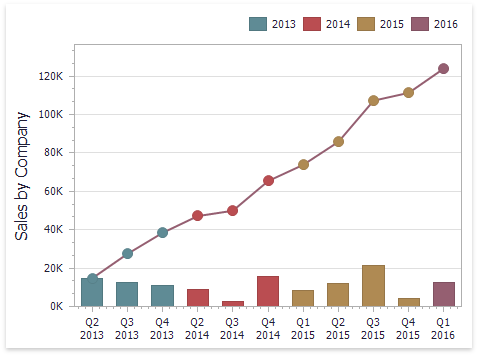The DevExpress Dashboard allows you to apply window calculations to values of the specified measure. The following calculation types are supported.

• Running Total - Allows you to calculate a cumulative total for a set of measure values.

Expression: RunningSum(Sum([Sales]))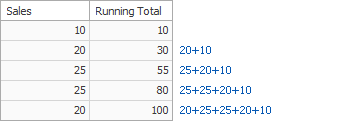• Moving Calculation - Allows you to apply a moving calculation, which uses neighboring values to calculate a total. Note that neighboring values are specified using offsets from the currently processed value.

Expression: WindowSum(Sum([Sales]), -1, 1)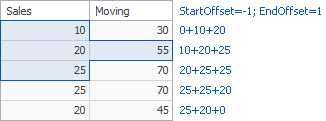• Difference - Allows you to compute differences between measure values.

Expression: Sum([Sales]) - Lookup(Sum([Sales]), -1)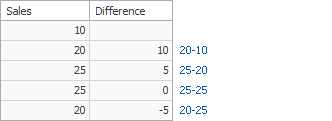• Percent of Total - Allows you to calculate a contribution of individual measure values to a total.

Expression: Sum([Sales])) / Total(Sum([Sales]))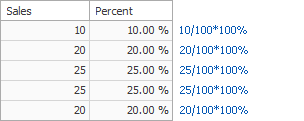• Rank - Allows you to rank values of the specified measure.

Expression: RankCompetition(Sum([Sales]), ‘asc’)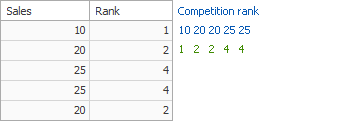Note

The dashboard control does not allow sorting by measures with applied Window Calculations since the calculation value can depend on the calculated element position.

## How to Use Window Calculations

The topic below show how to use window calculations in the WinForms Designer and on the web.